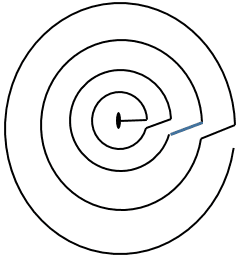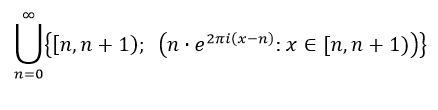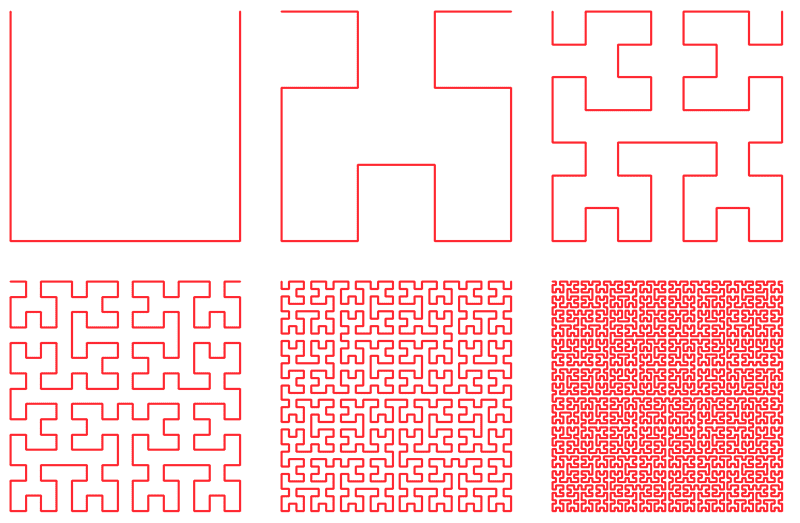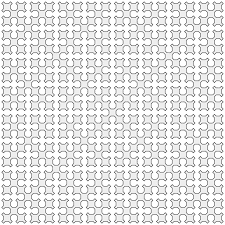# Why is this not a space-filling curve?

• I
Gold Member
TL;DR Summary
On a variation of the lexicographical order, but in polar coordinates (identifying angles with the same position in the plane), this would naively seem to be both a linear order (not amenable to a field structure!) and to cover the plane... but this would be too easy, so I am overlooking something basic....
On a plane with a selected origin point and a selected zero rotation direction, identify each point p with (rpp), where rp is the distance to the origin and θp is the angle in [0, 2π). Define an order ≤* between points p and q as b

p=*q if they are identical,
p <* q if
 rp < rq, or
 rp = rq & θp = θq
(that is, if the plane consists of concentric circles around the origin, then those on the inner circles are less than those on the outer circles, and inside a circle, the points at a smaller non-negative angle (mod 2π) are smaller than the points with a larger one).

Either what is wrong with this definition (and could it be easily fixed), why isn't it a linear order (yes, I know that it can't be made into a field structure), and/or why isn't it a space-filling curve?

SSequence
Seems like a linear-order (on ##\mathbb{R}^2##) to me. I don't remember the formal definitions fully well but, for example, denoting <* in your post by less we have the following as true:
---- ## \forall p \in \mathbb{R^2} \,\,\, [\neg \, less(p,p)] ##
---- ## \forall p,q \in \mathbb{R^2} \,\,\, [ \, p \neq q \rightarrow (less(p,q) \oplus less(q,p)) ] ##
---- ## \forall p,q,r \in \mathbb{R^2} \,\,\, [ (less(p,q)\,\, \land\,\, less(q,r)) \rightarrow less(p,r) ] ##

But as I understand the definition of curve is more complicated isn't it? That is, any arbitrary subset of ##\mathbb{R}^2## doesn't qualify as a curve. I don't know anything about this subject (the term "jordan curve" is likely relevant).

P.S.
On that note, if we consider a curve (in the sense of ordinary discourse ... without any "crossing") ##S \subset \mathbb{R}^2## then we can define a linear order on ##S## (based on how much distance we have traveled from "one end" to the "other end"). This is kind of obvious but still I thought I would mention it.

Last edited:
Gold Member

A side note: the term "partial order" has two definitions wandering around, one for "less", and one for "less than or equal to". Your answer showed you were testing you tested the order "less"; I originally meant the latter, leaving "p ≤* q : ⇔ p=*q or p <* q" implicit. But it doesn't matter, it comes out the same in the wash.

Anyway, yes, linear order is a partial order plus the additional axiom that any two elements are comparable
(p =*q or less (p,q) or less(q,p)). As far as I see, that also fits, no?

The idea that the definition of a curve might be relevant is interesting; as far as I can see, any continuous function (or its image from an interval) is a curve, no? (The site https://mathworld.wolfram.com/Curve.html gives three definitions). I have an intuition that such a function could be proven to exist (constructively or otherwise, as I am not a constructivist). But I will have to work on that, and post my results. (Watch this space.🌋). Thanks for the suggestion.

SSequence
Yeah there are lot of definitions for related terms. I do tend to get confused (or forget) the precise definitions/meanings of terms. The issue of totality of relation also seems to add to the number of definitions.

Anyway, yes, linear order is a partial order plus the additional axiom that any two elements are comparable
(p =*q or less (p,q) or less(q,p)). As far as I see, that also fits, no?
I think what you are saying is that the relation <* or ##less: \mathbb{R}^2 \times \mathbb{R}^2 \rightarrow \{0,1\}## must be total. I think so. This is also implied/covered by the first two statements (in logic symbols) in post#2.

Anyway, I know very little analysis (beyond very basics ... let alone any follow-up topics) so I can't add much to the discussion. Though I found an interesting link (these seem to come up on first or second page) while searching for "jordan curve theorem" (slightly tangent to thread topic though):
https://people.math.osu.edu/fiedorowicz.1/math655/Jordan.html
https://www.math.brown.edu/~res/MathNotes/jordan.pdf

The topic of space-filling curve also sound quite interesting.

Last edited:
Either what is wrong with this definition (and could it be easily fixed), why isn't it a linear order (yes, I know that it can't be made into a field structure), and/or why isn't it a space-filling curve?
You have defined an ordering, but where have you defined a curve?

Gold Member
Thanks for the links, SSequence. The curve, if it is indeed a curve, that I am referring to is of course not a Jordan curve.

Good question, Svein. I left this implicit, that the total order would be on R2 and by replacing <* by “close to”. I still haven’t got the corresponding function in a closed form, and I am not sure it’s possible, but perhaps as a series of curves which converge to the desired curve, as one does with the usual examples of space-filling curves, such as the Peano curve.. That is, one might start with something like this (gaps in each circle exaggerated, and diagram keeps going forever) and start shrinkingOr, somewhat sloppily described:and then head for the appropriate limits (needs work, but you get the idea, I hope).

Of course, the strict definition of space-filling curve is one which can fill the unit square – i.e., not filling all of space, but OK, one could limit this process to fill the circle + its interior of appropriate size… a circle, not a square, but since they are easy to transform one into another, this comes to the same thing.

BTW, you have defined a lattice (https://en.wikipedia.org/wiki/Lattice_(order)). For space-filling curves you have this:and this:Gold Member
Thanks for the nice drawing , saving the reader to go look up the reference to the Peano curve in my post.
I am not sure where it is that I defined a lattice. That it is a partial order is clear, but where did I define a join and a meet?

Thanks for the nice drawing , saving the reader to go look up the reference to the Peano curve in my post.
Nitpicking: The first curve is the first 6 iterations of a Hilbert curve. The other is a Sierpinski curve (at level 8?).

As to meet or infimum, the point (0, 0) (or possibly the whole class with r=0) is an obvious contender. You may need to extend your definition slightly to get a unique meet (consider two points with the same r but different Θ). Join or supremum - either define a maximum for r or use another metric (like r/(r+1)).

Gold Member
Thanks for the correction, Svein, on the drawings. Anyway, the idea is the idea of iterations, of a family of curves which converge to a space-filling curve.

I did not mean that the meet would be the infinum of the whole curve, but simply that the meet of two elements could be defined as the infimum of the set of the two elements. The meet in this curve being the origin would only apply to sets, one of which was the origin. For join, the curve could have a join without having a supremum for the curve, as the join only relates to sets of two elements at a time. No other metric is necessary to define a join.

I am not quite sure what you meant by having a metric "like r/(r+1)". Please explain.

Also, I have yet to understand the relevance of the lattice structure for this curve in order to determine whether it would qualify as a space-filling curve.

Also, I have yet to understand the relevance of the lattice structure for this curve in order to determine whether it would qualify as a space-filling curve.
Just an observation - not important.
I am not quite sure what you meant by having a metric "like r/(r+1)". Please explain.
This is a standard trick when distances go to infinity (when r→∞, r/(r+1)→1).

Again, you have not defined a space-filling curve (your drawing in post #6 has no obvious extension). Hint: Define iteration n of a curve as $r=e^{-\theta\cdot 2^{-n}}$ and check if it qualifies.

Gold Member
If I am graphing your suggestion correctly r=exp(-θ/2n) is a spiral that converges to a circle. It does not work as a space-filling curve. Thanks anyway for the suggestion.

your drawing in post #6 has no obvious extension
The drawing was meant as a heuristic indication, where the extension would start by extending the construction out to infinity, and then somehow make the radii of the circles converge so that the set of radii = the non-zero real line. A cardinality problem stops this from being straightforward, so I was wondering whether there was some way to fulfill this intuitive picture.